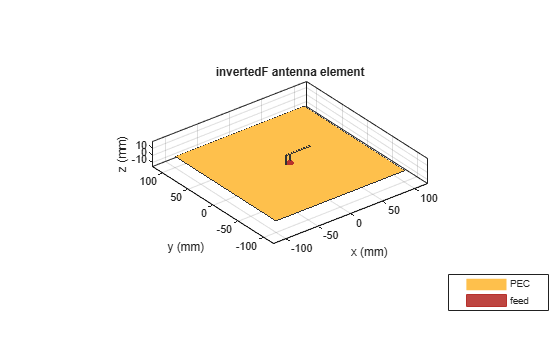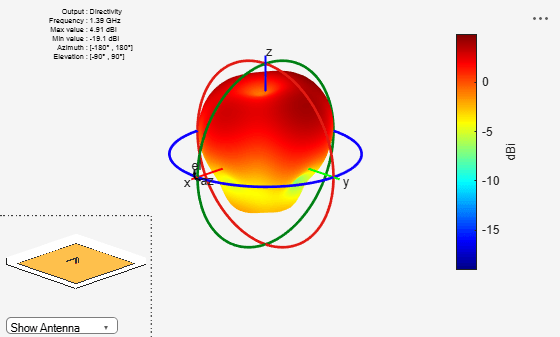# invertedF

Create inverted-F antenna over rectangular ground plane

## Description

The `invertedF` object is an inverted-F antenna mounted over a rectangular ground plane.

The width of the metal strip is related to the diameter of an equivalent cylinder by the equation

`$w=2d=4r$`

where:

• d is the diameter of equivalent cylinder

• r is the radius of equivalent cylinder

For a given cylinder radius, use the utility function `cylinder2strip` to calculate the equivalent width. The default inverted-F antenna is center-fed. The feed point coincides with the origin. The origin is located on the xy- plane.## Creation

### Syntax

``f = invertedF``
``f = invertedF(Name,Value)``

### Description

``` `f = invertedF` creates an inverted-F antenna mounted over a rectangular ground plane. By default, the dimensions are chosen for an operating frequency of 1.7 GHz.```

example

``` `f = invertedF(Name,Value)` creates an inverted-F antenna, with additional properties specified by one, or more name-value pair arguments. `Name` is the property name and `Value` is the corresponding value. You can specify several name-value pair arguments in any order as `Name1`, `Value1`, ..., `NameN`, `ValueN`. Properties not specified retain their default values.```

## Properties

expand all

Vertical element height along z-axis, specified a scalar in meters.

Example: `'Height',3`

Data Types: `double`

Strip width, specified as a scalar in meters.

Note

Strip width should be less than `'Height'`/4 and greater than `'Height'`/1001. 

Example: `'Width',0.05`

Data Types: `double`

Stub length from feed to open end, specified as a scalar in meters.

Example: `'LengthToOpenEnd',0.05`

Stub length from feed to shorting end, specified as a scalar in meters.

Example: `'LengthToShortEnd',0.0050`

Ground plane length along x-axis, specified as a scalar in meters. Setting `'GroundPlaneLength'` to Inf, uses the infinite ground plane technique for antenna analysis.

Example: `'GroundPlaneLength',4`

Data Types: `double`

Ground plane width along y-axis, specified as a scalar in meters. Setting `'GroundPlaneWidth'` to Inf, uses the infinite ground plane technique for antenna analysis.

Example: `'GroundPlaneWidth',2.5`

Data Types: `double`

Signed distance from center along length and width of ground plane, specified as a two-element vector.

Example: `'FeedOffset',[2 1]`

Data Types: `double`

Type of the metal used as a conductor, specified as a metal material object. You can choose any metal from the `MetalCatalog` or specify a metal of your choice. For more information, see `metal`. For more information on metal conductor meshing, see Meshing.

Example: ```m = metal('Copper'); 'Conductor',m```

Example: ```m = metal('Copper'); ant.Conductor = m```

Lumped elements added to the antenna feed, specified as a lumped element object. For more information, see `lumpedElement`.

Example: `'Load',lumpedelement`. `lumpedelement` is the object for the load created using `lumpedElement`.

Example: ```f.Load = lumpedElement('Impedance',75)```

Tilt angle of the antenna, specified as a scalar or vector with each element unit in degrees. For more information, see Rotate Antennas and Arrays.

Example: `'Tilt',90`

Example: `ant.Tilt = 90`

Example: `'Tilt',[90 90]`,`'TiltAxis',[0 1 0;0 1 1]` tilts the antenna at 90 degrees about the two axes defined by the vectors.

Note

The `wireStack` antenna object only accepts the dot method to change its properties.

Data Types: `double`

Tilt axis of the antenna, specified as:

• Three-element vector of Cartesian coordinates in meters. In this case, each coordinate in the vector starts at the origin and lies along the specified points on the X-, Y-, and Z-axes.

• Two points in space, each specified as three-element vectors of Cartesian coordinates. In this case, the antenna rotates around the line joining the two points in space.

• A string input describing simple rotations around one of the principal axes, 'X', 'Y', or 'Z'.

Example: `'TiltAxis',[0 1 0]`

Example: `'TiltAxis',[0 0 0;0 1 0]`

Example: `ant.TiltAxis = 'Z'`

Note

The `wireStack` antenna object only accepts the dot method to change its properties.

Data Types: `double`

## Object Functions

 `show` Display antenna or array structure; display shape as filled patch `info` Display information about antenna or array `axialRatio` Axial ratio of antenna `beamwidth` Beamwidth of antenna `charge` Charge distribution on metal or dielectric antenna or array surface `current` Current distribution on metal or dielectric antenna or array surface `design` Design prototype antenna or arrays for resonance around specified frequency `efficiency` Radiation efficiency of antenna `EHfields` Electric and magnetic fields of antennas; Embedded electric and magnetic fields of antenna element in arrays `impedance` Input impedance of antenna; scan impedance of array `mesh` Mesh properties of metal or dielectric antenna or array structure `meshconfig` Change mesh mode of antenna structure `optimize` Optimize antenna or array using SADEA optimizer `pattern` Radiation pattern and phase of antenna or array; Embedded pattern of antenna element in array `patternAzimuth` Azimuth pattern of antenna or array `patternElevation` Elevation pattern of antenna or array `rcs` Calculate and plot radar cross section (RCS) of platform, antenna, or array `returnLoss` Return loss of antenna; scan return loss of array `sparameters` Calculate S-parameter for antenna and antenna array objects `vswr` Voltage standing wave ratio of antenna

## Examples

collapse all

Create and view an inverted-F antenna with 14 mm height over a ground plane of dimensions 200 mm-by-200 mm.

```f = invertedF('Height',14e-3, 'GroundPlaneLength',200e-3, ... 'GroundPlaneWidth',200e-3); show(f)```This example shows you how to plot the radiation pattern of an inverted-F antenna for a frequency of 1.3 GHz.

```f = invertedF('Height',14e-3, 'GroundPlaneLength', 200e-3, ... 'GroundPlaneWidth', 200e-3); pattern(f,1.39e9)```Balanis, C.A. Antenna Theory. Analysis and Design, 3rd Ed. New York: Wiley, 2005.

 Volakis, John. Antenna Engineering Handbook, 4th Ed. New York: Mcgraw-Hill, 2007.